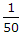Civil Engineering - Highway Engineering

Exercise :: Highway Engineering - Section 6

41.

Roadway width for a National highways and State highways (two-lanes) is

 A. 12 m B. 9 m C. 9.5 m D. 15 m

Explanation:

No answer description available for this question. Let us discuss.

42.

The distance travelled by revolving the wheel of a vehicle more than its circumferential movement, is known as

 A. slip B. skid C. neither (a) nor (b) D. both (a) and (b)

Explanation:

No answer description available for this question. Let us discuss.

43.

If the difference in elevation of an edge of the pavement 9 m wide and its crown is 15 cm, the camber of the pavement, is

 A. 1 in 60 B. 1 in 45 C. 1 in 30 D. 1 in 15 E. 1 in 7.5

Explanation:

No answer description available for this question. Let us discuss.

44.

If degree of a road curve is defined by assuming the standard length of an arc as 30 metres, the radius of 1° curve is equal

 A. 1719 m B. 1146 m C. 1046 m D. 1619 m E. 1573 m

Explanation:

No answer description available for this question. Let us discuss.

45.

For calculating the tractive force along an upgrade of an asphalt road, the most probable value of the co-efficient of traction resistance μ is assumed

 A.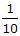B.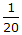C.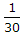D.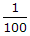E.